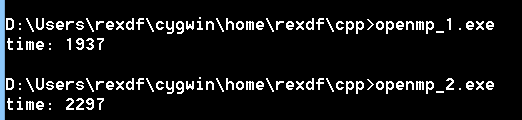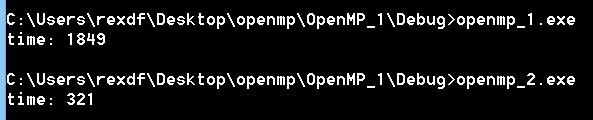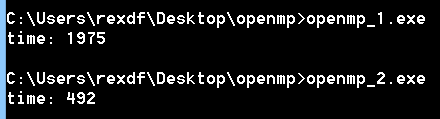# OpenMP在win上面的编译

#include <iostream>
#include <time.h>
void test()
{
int a = 0;
for (int i=0;i<100000000;i++)
a++;
}
int main()
{
clock_t t1 = clock();
for (int i=0;i<8;i++)
test();
clock_t t2 = clock();
std::cout<<"time: "<<t2-t1<<std::endl;
}


#include <iostream>
#include <time.h>
void test()
{
int a = 0;
for (int i=0;i<100000000;i++)
a++;
}
int main()
{
clock_t t1 = clock();
#pragma omp parallel for
for (int i=0;i<8;i++)
test();
clock_t t2 = clock();
std::cout<<"time: "<<t2-t1<<std::endl;
}